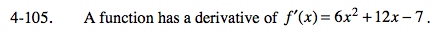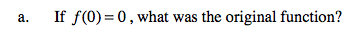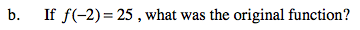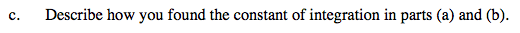### Home > CALC > Chapter 4 > Lesson 4.3.1 > Problem4-105

4-105.
1. A function has a derivative of f ′(x) = 6x2 + 12x − 7. Homework Help ✎

1. If f(0) = 0, what was the original function?

2. If f(−2) = 25, what was the original function?

3. Describe how you found the constant of integration in parts (a) and (b).Solve for C by evaluating f(x) at f(0) = 0.

Note: you could also use the expressionf(x) = 2x³ + 6x² − 7x + C
Evaluate the given point to solve for C.

Note: you could also use the expressionRefer to hints in part (a) and (b).